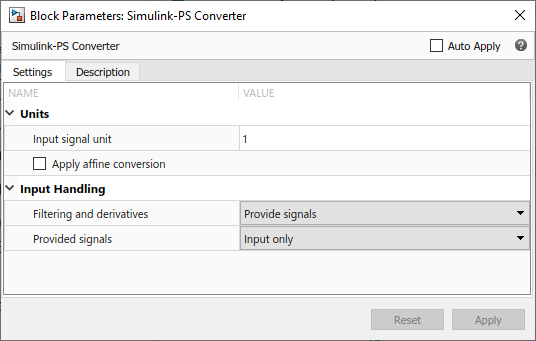## Filtering Input Signals and Providing Time Derivatives

You may need to provide time derivatives of some of the input signals, especially if you use an explicit solver. One way of providing the necessary input derivatives is by filtering the input through a low-pass filter. Input filtering makes the input signal smoother and generally improves model performance. The additional benefit is that the Simscape™ engine computes the time derivatives of the filtered input. The first-order filter provides one derivative, while the second-order filter provides the first and second derivatives. If you use input filtering, it is very important to select the appropriate value for the filter time constant.

The filter time constant controls the filtering of the input signal. The filtered input follows the true input but is smoothed, with a lag on the order of the time constant that you choose. Set the time constant to a value no larger than the smallest time interval in the system that interests you. If you choose a very small time constant, the filtered input signal is closer to the true input signal. However, this filtered input signal increases the stiffness of the system and slows the simulation.

Instead of using input filtering, you can provide time derivatives for the input signal directly, as additional Simulink® signals.

For piecewise-constant signals, you can also explicitly set the input derivatives to zero.

You can control the way you provide time derivatives for each input signal by configuring the Simulink-PS Converter block connected to that input signal:

1. Open the Simulink-PS Converter block dialog box.

2. Expand the Input Handling section.3. When you add a new Simulink-PS Converter block to your model, the default input handling options are `Provide signals` and `Input only`, and the block has one Simulink input port and one physical signal output port.

4. To turn on input filtering, set the Filtering and derivatives parameter to ```Filter input, derivatives calculated```. Select the first-order or second-order filter, by using the Input filtering order parameter, and set the appropriate Input filtering time constant (in seconds) parameter value for your model.

5. To avoid filtering the input signal, keep the Filtering and derivatives parameter as `Provide signals`. Then set the Provided signals parameter value:

• `Input and first derivative` — If you select this option, an additional Simulink input port appears on the Simulink-PS Converter block, to let you connect the signal providing input derivative.

• `Input and first two derivatives` — If you select this option, two additional Simulink input ports appear on the Simulink-PS Converter block, to let you connect the signals providing input derivatives.

6. Finally, if your input signal is piecewise constant (such as step), you can also explicitly set the input derivatives to zero by selecting the ```Zero derivatives (piecewise constant)``` value for the Filtering and derivatives parameter.